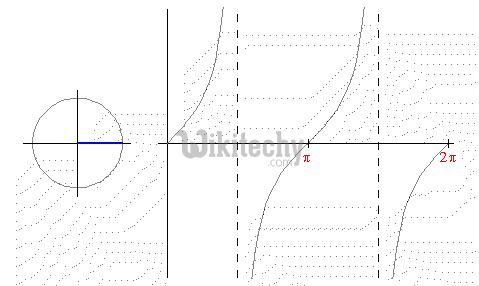# pig tutorial - apache pig tutorial - Apache Pig - TAN() - pig latin - apache pig - pig hadoop

## What is TAN() function?

• Tangent (tan) function is a Trigonometry function.
• In a right triangle, the tangent of an angle is the length of the opposite side divided by the length of the adjacent side.
• Sine, Cosine and Tangent are the main functions used in Trigonometry and are based on a Right-Angled Triangle.
• Before getting stuck into the functions, it helps to give a name to each side of a right triangle: "Opposite" is opposite to the angle θ "Adjacent" is adjacent (next to) to the angle θ.Learn Apache Pig - Apache Pig tutorial - tangent - Apache Pig examples - Apache Pig programs

## TAN() function in Apache Pig

• The TAN() function is used to calculate the trigonometric tangent of a given expression (angle).

## Syntax

• Ensure that we have a file named wikitechy_math.txt in the HDFS directory /pig_data/. This file contains integer and floating point values as given below.

wikitechy_math.txt

• We have loaded this file into Pig with a relation named math_data as given below.
• Now you can generate the tan values of the contents of the wikitechy_math.txt file using TAN() function as given below.

## Verification

• Verify the contents of the relation using the Dump operator as given below.

## Output

• The above statement stores the result in the relation named tan_data.